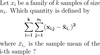Level 30 Level 32
Level 31

## Ignore words

Check the boxes below to ignore/unignore words, then click save at the bottom. Ignored words will never appear in any learning session.

Ignore?
Likelihood ratio test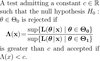Neyman-Pearson lemma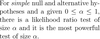Quantile function / Inverse CDF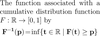Sample mean (x̄)Biased sample variance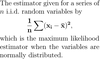Unbiased sample variance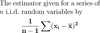Sample standard deviation (s)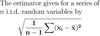Standard error of the mean (SEM)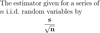Finite population correction (FPC)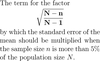Standard scorePooled variance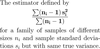Total sum of squares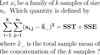Sum of squares of treatments / SST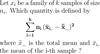Sum of squares of error / SSE